#### Semi-algebraic decomposition

As stated in Section 6.3.1, motion planning inside of each cell in a complex should be trivial. To solve the decision and quantifier-elimination problems, a cell decomposition was developed for which these problems become trivial in each cell. The decomposition is designed so that only a single point in each cell needs to be checked to solve the decision problem.

The semi-algebraic set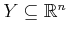that is expressed with (6.15) is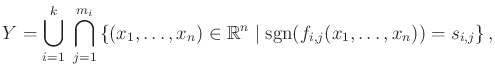(6.16)

in which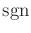is the sign function, and each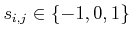, which is the range of. Once again the nice relationship between set-theory and logic, which was described in Section 3.1, appears here. We convert from a set-theoretic description to a logical predicate by changing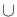and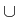to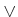and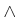, respectively.

Let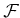denote the set of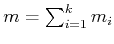polynomials that appear in (6.16). A sign assignment with respect tois a vector-valued function,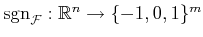. Each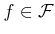has a corresponding position in the sign assignment vector. At this position, the sign,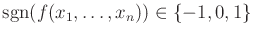, appears. A semi-algebraic decomposition is a partition of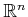into a finite set of connected regions that are each sign invariant. This means that inside of each region,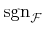must remain constant. The regions will not be called cells because a semi-algebraic decomposition is not necessarily a singular complex as defined in Section 6.3.1; the regions here may contain holes.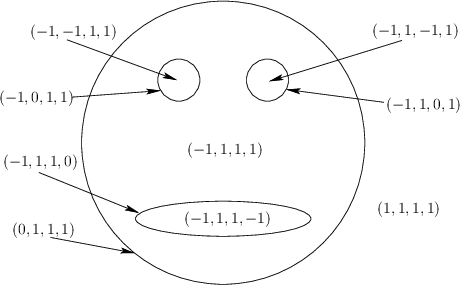Example 6..4 (Sign assignment)   Recall Example 3.1 and Figure 3.4 from Section 3.1.2. Figure 3.4a shows a sign assignment for a case in which there is only one polynomial,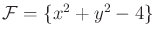. The sign assignment is defined as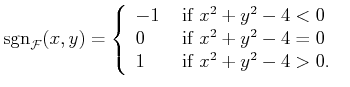(6.17)

Now consider the sign assignment, shown in Figure 6.30 for the gingerbread face of Figure 3.4b. The polynomials of the semi-algebraic model are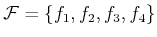, as defined in Example 3.1. In order, these are the head,'' left eye,'' right eye,'' and mouth.'' The sign assignment produces a four-dimensional vector of signs. Note that if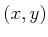lies on one of the zeros of a polynomial in, then a 0 appears in the sign assignment. If the curves of two or more of the polynomials had intersected, then the sign assignment would produce more than one 0 at the intersection points.

For the semi-algebraic decomposition for the gingerbread face in Figure 6.30, there are nine regions. Five 2D regions correspond to: 1) being outside of the face, 2)inside of the left eye, 3) inside of the right eye, 4) inside of the mouth, and 5) inside of the face but outside of the mouth and eyes. There are four 1D regions, each of which corresponds to points that lie on one of the zero sets of a polynomial. The resulting decomposition is not a singular complex because the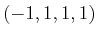region contains three holes.A decomposition such as the one in Figure 6.30 would not be very useful for motion planning because of the holes in the regions. Further refinement is needed for motion planning, which is fortunately produced by cylindrical algebraic decomposition. On the other hand, any semi-algebraic decomposition is quite useful for solving the decision problem. Only one point needs to be checked inside of each region to determine whether some Tarski sentence that has no free variables is true. Why? If the polynomial signs cannot change over some region, then the TRUE/FALSE value of the corresponding logical predicate,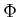, cannot change. Therefore, it sufficient only to check one point per sign-invariant region.

Steven M LaValle 2020-08-14